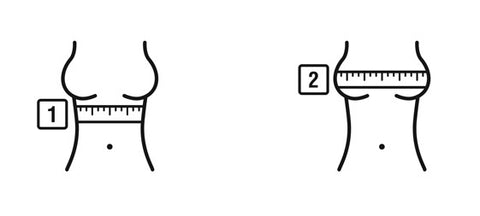##### (28-30) (32-34) (36-38)

Knowing your band size is essential to a good fit.

# EZ-Fit method

### The numbers - like 28, 30, 32, 34, 36, 38, are band sizes –  The band is the part of the bra that goes around our rib cage

Measure under the Bust to determine band measurement
Measure around directly under bust  &  around the rib cage round to nearest whole number to determine band size
If you measure your rib cage as 29 inches around
then your band size is 30
If you measure your rib cage as 27 inches around
then your band size is 28.
If you measure your rib cage as 34 inches around
then your band size is 34
If you measure your rib cage as 30 inches around
then your band size is 30
If you measure your rib cage as 31 inches
then your band size is 32
If you measure your rib cage as 33 inches around
then your band size is 34
If you measure your rib cage as 35 inches around
then your band size is 36.
If you measure your rib cage as 36 inches around
then your band size is 36
If you measure your rib cage as 37 inches around
then your band size is 38

*Band measurements always round up to nearest even number

## (28-30) (32-34) (36-38)### Band measurement is compared to Full bust measurement to determine bra cup size Measure across the Bust to determine cup size - measure the fullest part of the bust holding tape parallel with the floor to determine cup sizeBUST measurement - BAND SIZE  =  CUP SIZE inches 1 inch = A cup, 2 inch = B cup, 3 inch = C cup, 4 inch = D cup, 5 inch = DD cup What do the letters mean?The letters - like A, B, C, D, DD,- stand for cup sizes.

SOME EXAMPLES

A full bust measurement is compared to a band measurement to determine bra cup size.

A full-bust measurement of 35
is 5 inches larger than a
30 band measurement.
35 - 30 = 5 inches
These 5 inches are equivalent to a “DD” cup size; and a final bra size of 30DD.
1 inch = A cup,  2 inch = B cup, 3 inch = C cup, 4 inch = D cup, 5 inch = DD cup, 6 inch = DDD cup

A full bust measurement is compared to a band measurement to determine bra cup size.

#### A full-bust measurement of 36 is 4 inches larger than a32 band measurement36 - 32 = 4 inches These 4 inches are equivalent to a “D” cup size; and a final bra size of 32D.1 inch = A cup,  2 inch = B cup, 3 inch = C cup, 4 inch = D cup, 5 inch = DD cup, 6 inch = DDD cup